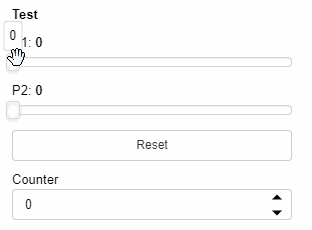# How to set value of the widget without executing watchers?

I have a function(heavy computation) that depends from 4 Widgets(IntSlider, FloatSlider, FloatInput, FloatSlider).
For each widget I add watcher to this function.

But now, I have a button that should set them to some global values. So I need to change all widgets values at once and call function only once.
So I need update all widgets value without executing watchers and then execute my function.
Do you have any suggestions how to implement it?

``````import param
import panel as pn
pn.extension()

class Test(param.Parameterized):

p1 = param.Number(default=0, bounds=(0,10))
p2 = param.Number(default=0, bounds=(0,10))
reset = param.Event()
counter = param.Integer(default=0)

@param.depends("p1", "p2", watch=True)
def heavy_function(self):
self.counter += 1

@param.depends("reset", watch=True)
def _reset(self):
with param.batch_watch(self):
self.p1 = 0
self.p2 = 0
``````1 Like

In the example you posted you could also more simply just do:

``````self.param.set_param(p1=0, p2=0)
``````

However I think @Illia was not necessarily using a param based approach so it would be great to know what exactly you are trying before I suggest something.

2 Likes